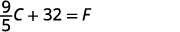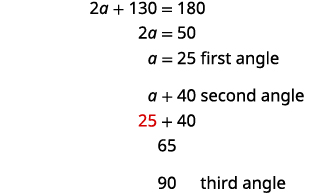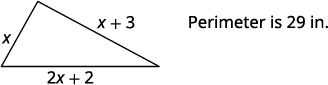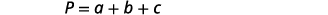# 2.4：求解特定变量的公式

•• OpenStax
• OpenStax
$$\newcommand{\vecs}{\overset { \rightharpoonup} {\mathbf{#1}} }$$ $$\newcommand{\vecd}{\overset{-\!-\!\rightharpoonup}{\vphantom{a}\smash {#1}}}$$$$\newcommand{\id}{\mathrm{id}}$$ $$\newcommand{\Span}{\mathrm{span}}$$ $$\newcommand{\kernel}{\mathrm{null}\,}$$ $$\newcommand{\range}{\mathrm{range}\,}$$ $$\newcommand{\RealPart}{\mathrm{Re}}$$ $$\newcommand{\ImaginaryPart}{\mathrm{Im}}$$ $$\newcommand{\Argument}{\mathrm{Arg}}$$ $$\newcommand{\norm}{\| #1 \|}$$ $$\newcommand{\inner}{\langle #1, #2 \rangle}$$ $$\newcommand{\Span}{\mathrm{span}}$$ $$\newcommand{\id}{\mathrm{id}}$$ $$\newcommand{\Span}{\mathrm{span}}$$ $$\newcommand{\kernel}{\mathrm{null}\,}$$ $$\newcommand{\range}{\mathrm{range}\,}$$ $$\newcommand{\RealPart}{\mathrm{Re}}$$ $$\newcommand{\ImaginaryPart}{\mathrm{Im}}$$ $$\newcommand{\Argument}{\mathrm{Arg}}$$ $$\newcommand{\norm}{\| #1 \|}$$ $$\newcommand{\inner}{\langle #1, #2 \rangle}$$ $$\newcommand{\Span}{\mathrm{span}}$$$$\newcommand{\AA}{\unicode[.8,0]{x212B}}$$

##### 学习目标

• 求解特定变量的公式
• 使用公式求解几何应用程序

1. 评估$$2(x+3)$$时间$$x=5$$
如果你错过了这个问题，请查看 [链接]
2. 矩形的长度比宽度小三个。 让 w 表示宽度。 为矩形的长度写一个表达式。
如果你错过了这个问题，请查看 [链接]
3. 评估$$\frac{1}{2}bh$$何时$$b=14$$$$h=9$$
如果你错过了这个问题，请查看 [链接]

#### 求解特定变量的公式

##### 示例$$\PageIndex{1}$$

 写下公式。移除右边的分数。简化。将两边除以$$πr^2$$。##### 示例$$\PageIndex{2}$$:

$$b=\frac{2A}{h}$$

##### 示例$$\PageIndex{3}$$:

$$h=\frac{2A}{b}$$

##### 示例$$\PageIndex{4}$$

 写下公式。移除右边的分数。简化。在两边加上 32。##### 示例$$\PageIndex{5}$$:

$$C=\frac{5}{9}(F−32)$$

##### 示例$$\PageIndex{6}$$:

$$b=\frac{2A−Bh}{h}$$

##### 示例$$\PageIndex{7}$$

 写下公式。通过$$2πr^2$$从两边减去来分离该$$h$$项。简化。$$h$$通过将两边除以来求解$$2πr.$$简化。##### 示例$$\PageIndex{8}$$:

$$t=\frac{A−P}{Pr}$$

##### 示例$$\PageIndex{9}$$:

$$r=\frac{A−P}{Pt}$$

 我们将在方程的一$$y$$侧进行隔离。$$6x$$从两边减去以将术语与之隔离开来$$y$$。简化。将两边除$$7$$以得出系数为$$y$$一。简化。$$y=\frac{9−4x}{7}$$

##### 示例$$\PageIndex{12}$$

$$y=\frac{1−5x}{8}$$

#### 使用公式求解几何应用程序

##### 求解几何应用程序。
1. 阅读问题并确保所有文字和想法都被理解。
2. 确定你在找什么。
3. 通过选择一个变量来表示我们要找的东西来@@ 命名它。 画出图形并用给定的信息贴上标签。
4. 通过编写适合情况的公式或模型将其@@ 转换为方程。 在给定的信息中替换。
5. 使用良好的代数技巧求@@ 方程。
6. 检查问题中的答案并确保答案合理。
7. 用完整的句子@@ 回答问题。##### 示例$$\PageIndex{13}$$

 第 1 步。 阅读问题。 第 2 步。 确定你在找什么。 三角形的高度 第 3 步。 姓名。 选择一个变量来表示它。 让$$h=$$高度。 画出图形并用给定的信息贴上标签。 面积 = 126 平方英寸第 4 步。 翻译。 写出相应的公式。 $$A=\frac{1}{2}bh$$ 在给定的信息中替换。 $$126=\frac{1}{2}·18·h$$ 第 5 步。 求解方程。 $$126=9h$$ 将两边除以 9。 $$14=h$$ 第 6 步。 查看。 \begin{align*} A &= \frac{1}{2}bh \\126 & \stackrel{?}{=} 12·18·14 \\ 126 &=126✓ \end{align*} 第 7 步。 回答问题。 三角形的高度为$$14$$英寸。

##### 示例$$\PageIndex{16}$$:

 第 1 步。 阅读问题。 第 2 步。 确定你在找什么。 所有三个角度的测量 第 3 步。 姓名。 选择一个变量来表示它。 \begin{align*} \text{Let }a \; & = \; \mathrm{1^{st} \; angle.} \\ a+40 &= \mathrm{2^{nd} \; angle} \\90 &= \mathrm{3^{rd} \; angle \; (the \; right \; angle)} \end{align*} 画出图形并用给定的信息贴上标签。第 4 步。 翻译。 写出相应的公式。代入配方。第 5 步。 求解方程。第 6 步。 查看。 \begin{align*} 25+65+90 & \stackrel{?}{=} 180\\ 180 &= 180✓ \end{align*} 第 7 步。 回答问题。 三个角度测量$$25°,\;65°$$，和$$90°$$。

##### 毕达哥拉斯定理##### 示例$$\PageIndex{19}$$:第 1 步。 阅读问题。 第 2 步。 确定你在找什么。 三角形腿的长度 第 3 步。 姓名。 选择一个变量来表示它。 让$$a$$ = 三角形的腿。 在 a 面贴标签第 4 步。 翻译。 写出相应的公式。 替代。 \begin{align*}a^2+b^2 &=c ^2 \\ a^2+12^2 &=13^2 \end{align*} 第 5 步。 求解方程。 分离变量项。 使用平方根的定义。 简化。 \begin{align*} a^2+144 &= 169 \\ a^2 &= 25 \\ a &= \sqrt{25} \\ a&=5 \end{align*} 第 6 步。 查看。第 7 步。 回答问题。 腿的长度是$$5$$。
##### 示例$$\PageIndex{20}$$:##### 示例$$\PageIndex{21}$$:##### 示例$$\PageIndex{22}$$:

 第 1 步。 阅读问题。 第 2 步。 确定我们在寻找什么。 长度和宽度 第 3 步。 姓名。 选择一个变量来表示宽度。 长度为六倍是宽度的两倍。 让$$w=$$宽度。 $$2w+6=$$长度$$P=96$$厘米 第 4 步。 翻译。 写出相应的公式。在给定的信息中替换。第 5 步。 求解方程。第 6 步。 查看。\begin{align*} P &=2L+2W \\ 96 & \stackrel{?}{=}2·34+2·14 \\ 96 &=96✓ \end{align*} 第 7 步。 回答问题。 长度为$$34$$厘米，宽度为$$14$$厘米

##### 示例$$\PageIndex{25}$$:

 第 1 步。 阅读问题。 第 2 步。 确定我们在寻找什么。 三角形三边的长度 第 3 步。 姓名。 选择一个变量来表示第一边的长度。 \begin{align*} \mathrm{Let \;}x \;& \mathrm{= \; length \; of \;1^{st} \;side.} \\ x+3 \; &= \; \mathrm{length \; of \; 2^{nd} \; side} \\ 2x+2 \; &= \; \mathrm{length \; of \;3^{rd} \; side} \end{align*}第 4 步。 翻译。 写出相应的公式。 在给定的信息中替换。第 5 步。 求解方程。第 6 步。 查看。\begin{align*} 29 & \stackrel{?}{=}6+9+14 \\ 29 &= 29✓ \end{align*} 第 7 步。 回答问题。 三角形边的长度为$$6$$$$9$$、和$$14$$英寸。

##### 示例$$\PageIndex{28}$$:第 1 步。 阅读问题。 第 2 步。 确定我们在寻找什么。 足球场的长度和宽度 第 3 步。 姓名。 选择一个变量来表示它。 长度比宽度多 40 英尺。 画出图形并用给定的信息贴上标签。 假设 w = 宽度。 $$w+40=$$长度第 4 步。 翻译。 写出相应的公式并替代。第 5 步。 求解方程。 第 6 步。 查看。 \begin{align*} P &=2L+2W \\ 360 & \stackrel{?}{=} 2(110)+2(70) \\360 &=360✓ \end{align*}第 7 步。 回答问题。 足球场的长度是$$110$$英尺，宽度是$$70$$英尺。

##### 示例$$\PageIndex{31}$$:第 1 步。 阅读问题。 第 2 步。 确定我们在寻找什么。 支架应安装到角落的距离 第 3 步。 姓名。 选择一个变量来表示它。 画出图形并用给定的信息贴上标签。 让$$x=$$距离角落保持距离。第 4 步。 翻译。 写出相应的公式并替代。 $$a^2+b^2=c^2$$$$x^2+x^2=10^2$$ 第 5 步。 求解方程。 隔离变量。 使用平方根的定义。 简化。 近似到最接近的十分之一。 \begin{align*} 2x^2 &= 100 \\ \\ x^2 &=50 \\ \\ x &= \sqrt{50} \\ \\ x &≈7.1 \end{align*} 第 6 步。 查看。 \begin{align*} a^2+b^2 &= c^2 \\ (7.1)^2+(7.1)^2 &≈10^2 \; \;\;\;\; \text{Yes.} \end{align*} 第 7 步。 回答问题。 开尔文应该将每块木头固定在距离角落大约 7.1 英寸的地方。
##### 示例$$\PageIndex{32}$$:##### 示例$$\PageIndex{33}$$:• 求解文字方程

## 关键概念

• 如何解决几何应用程序
1. 阅读问题并确保所有文字和想法都被理解。
2. 确定你在找什么。
3. 通过选择一个变量来表示你要找的东西来@@ 命名它。 画出图形并用给定的信息贴上标签。
4. 通过编写适合情况的公式或模型将其@@ 转换为方程。 在给定的信息中替换。
5. 使用良好的代数技巧求@@ 方程。
6. 检查问题中的答案并确保答案合理。
7. 用完整的句子@@ 回答问题。
• 毕达哥拉斯定理
• 在任何直角三角形中，ab 是腿的长度，c 是斜边的长度，两条腿长度的平方和等于斜边长度的平方。